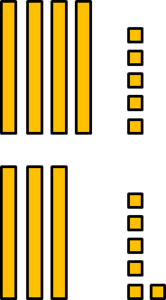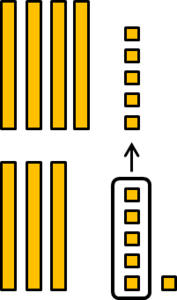# Ford and Logan Add 45+36

Alignments to Content Standards: 1.NBT.C.4 2.NBT.B.5

Actions

Part One:  Solve the problem and explain your thinking.

45+36Part Two:  Ford and Logan each solved the problem using a different strategy.

1. How did Ford solve the problem?  Will his strategy always work?
2. How did Logan solve the problem?  Will her strategy always work?
3. How are their strategies similar or different?
4. How was your strategy similar or different than Ford or Logan’s?

Ford’s thinking:I Counted the tens first, so 10, 20, 30, 40, 50, 60, 70.

Then I counted the ones, 71, 72, 73, 74, 75, 76, 77, 78, 79, 80, 81.

So 45+36=81.

Logan’s thinking:First I broke 36 into 30+1+5.

Then I gave 5 from 36 to the 45 to make 50 because 50 is a friendly number.

Then I added 30+50 to make 80.  Then I added 1 to 80 to get 81.

So 45+36=81.

## IM Commentary

This task was designed to give students opportunities to solve a problem and analyze other student’s solutions while working on adding two-digit numbers. Students will explore strategies based on place value and properties of operations as they solve 45+36 and explore two additional solution strategies. Through a class discussion, the teacher can support students in understanding that numbers can be decomposed by place value and like units can be combined (tens added with tens, ones added with ones). Two representations are provided, connecting concrete models or drawings with a more abstract representation using numbers.  As part of this task, students will engage in MP3,  construct viable arguments and critique the reasoning of others, as they analyze Ford and Logan's strategies, make sense of their strategies, and evaluate if they are valid.

This task goes a little beyond the expectations of the grade 1 standard to which it is aligned, but uses the types of reasoning expected in grade 1 and early grade 2, and does not reflect the fluency expectations for the end of grade 2. Thus this task represents the work students do as they transition from grade 1 to grade 2.

## Solution

Part One:  45+36=81

Part Two:  Answers will vary.  Some possibilities are listed below.

1. Ford used blocks representing the tens and ones to represent 45 and 36.  He counted the tens first and then he counted the ones.  His strategy will always work because you can represent numbers with place value blocks and then count them.
2. Logan broke apart 45 and 36 into tens and ones, 40 and 5, and 30 and 6. She then gave 5 ones from 36 to 45 to make 50, a decade number. She then added the remaining 3 tens to 50 to make 80 and the remaining 1 to make 81. Her method depends on the particular numbers, although it is a strategy that often works.
3. Their strategies are similar because they both used place value, thinking about each addend and the tens and ones that composed it. Their strategies are different in the way they decomposed and recomposed values. Ford combined like units, working with tens and ones separately, then combining them. Logan maintained one addend, 45, then combined ones from the other addend to make a decade number. She then worked with the remaining numbers first adding tens, then ones.